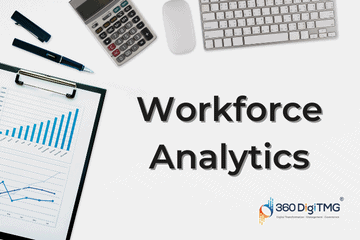Home / Blog / Data Science / Stock Market Analysis and Prediction using Machine Learning Models

# Stock Market Analysis and Prediction using Machine Learning Models

• June 26, 2023
• 3693
• 66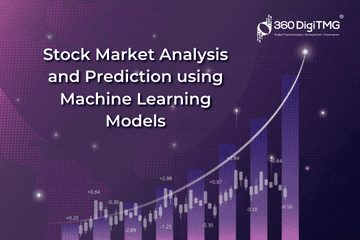### Meet the Author : Mr. Bharani Kumar

Bharani Kumar Depuru is a well known IT personality from Hyderabad. He is the Founder and Director of Innodatatics Pvt Ltd and 360DigiTMG. Bharani Kumar is an IIT and ISB alumni with more than 17 years of experience, he held prominent positions in the IT elites like HSBC, ITC Infotech, Infosys, and Deloitte. He is a prevalent IT consultant specializing in Industrial Revolution 4.0 implementation, Data Analytics practice setup, Artificial Intelligence, Big Data Analytics, Industrial IoT, Business Intelligence and Business Management. Bharani Kumar is also the chief trainer at 360DigiTMG with more than Ten years of experience and has been making the IT transition journey easy for his students. 360DigiTMG is at the forefront of delivering quality education, thereby bridging the gap between academia and industry.

## What are stock market analysis and prediction?

The process of attempting to determine the longer-term value of a company's shares or other financial instrument traded on an exchange is known as stock market prediction. Successfully predicting the price of a stock might result in huge rewards.

Machine learning (ML) is a technique that gives systems the freedom to learn on their own by interacting with the actual world and extrapolating from examples without being explicitly coded, as is the case with rule-based programming.

yourself a promising career in Machine Learning Training Center in Chennai by enrolling in the Machine Learning Program offered by 360DigiTMG.

A huge variety of crucial applications can benefit greatly from machine learning. Regression (LR) is a fundamental machine learning method for obtaining a linear trend. However, Support Vector Machines (SVMs) include sophisticated properties including great predictability and accuracy. Comparing the accuracy of the methods, and highlighting the advantages and disadvantages of utilising various strategies to forecast results.

One of the foremost important tasks in ML is to predict, with high accuracy and speed, the trend and therefore the results for any given dataset. Before the time of computing (AI) and ML, predictions were done manually by a statistician who would plot graphs and use mathematical methods and models to watch trends. One of these methods was to fit a line of the shape y = mx + c to a graph such that the road passes through the most number of knowledge points of the given dataset. Mathematically speaking, when plotting the values of the dataset on a graph, fit a line through the points such that the square of the space between each point and also the line is minimum. This line, called the hypothesis, is employed to predict the y value for any given x. This prediction technique is named regression toward the mean and also the formula used is named the smallest amount Squares method. This method is widely known to statisticians and has also been used collectively in the fundamental concepts of ML.

### Learn the core concepts of Data Science Course video on Youtube:

Simple regression's hypothesis function has a general structure similar to the equation of a line y = h (x) = 0+1x... (1). H(x) is given the values 0 and 1 to force the predicted output y. It should be noted that the challenge is to push a variety of 0 and 1 values to find values that provide the best "fit" or the most representational "straight line" across the data points mapped on the x-y plane. The cost function, which takes the mean of all the outputs of the hypothesis with inputs from x's compared to the specific output y's, is used to assess the correctness of our hypothesis. This function is frequently referred to as the Mean Squared Error function.

## Methodology and System setup

Environment

Python Programming and R may be considered to perform the prediction using the above two Machine Learning Models. During this work, RStudio is employed to analyze the error function and predict the acceptable model for the dataset

Time Series Forecasting

A statistic can be a set of data points that have been arranged chronologically (or listed, enumerated, or graphed). A statistic is most often a series of measurements made at successive, evenly spaced moments in time. As a result, it is a collection of discrete-time data. The statistical analysis includes techniques for deriving useful statistics and other informational features from statistical data. Statistical forecasting is the process of using a model to make predictions about the future that are supported by data from the past. The prediction might be a component of statistical inference in statistics.

Sliding-Window Method
In statistical prediction, the statistics are typically expanded into three or higher-dimensional spaces to use the data that's underlying them. Given a sequence of numbers for a statistical dataset, the information may be restructured to appear as sort of a supervised learning problem. This may be done by using previous time steps as input variables and using the subsequent time step as the output variable.

What are stock market analysis and prediction?

Also, check this Machine Learning with Python Course in Pune to start a career in Machine Learning.

The process of attempting to determine the longer-term value of a company's shares or other financial instrument traded on an exchange is known as stock market prediction. Successfully predicting the price of a stock might result in huge rewards.

Machine learning (ML) is a technique that gives systems the freedom to learn on their own by interacting with the actual world and extrapolating from examples without being explicitly coded, as is the case with rule-based programming.

Machine Learning can play a key role in an exceedingly wide selection of critical applications. In machine learning, regression (LR) could be a basic technique by which a linear trend is obtained. But Support Vector Machines (SVMs) have advanced features like high accuracy and predictability. Concluding with the pros and cons of using these techniques to predict values and compare the accuracy of the methods.

Predicting the trend and consequently the outcomes for any given dataset with high accuracy and speed is one of the most crucial challenges in machine learning (ML). A statistician would manually make forecasts before computing (AI and ML) by plotting graphs and using mathematical techniques and models to track trends.

One of these methods was to fit a line of the shape y = mx + c to a graph such that the road passes through the most number of knowledge points of the given dataset. Mathematically speaking, when plotting the values of the dataset on a graph, fit a line through the points such that the square of the space between each point and also the line is minimum. This line, called the hypothesis, is employed to predict the y value for any given x. This prediction technique is named regression toward the mean and also the formula used is named the smallest amount Squares method. This method is widely known to statisticians and has also been used collectively in the fundamental concepts of ML.

The general structure of the simple regression hypothesis function is identical to the equation of a line y = h(x) = 0+1x... (1). H(x) is given the values 0 and 1 to force the predicted output y. It should be noted that the challenge is to push a variety of 0 and 1 values to find values that provide the best "fit" or the most representational "straight line" across the data points mapped on the x-y plane. The cost function, which takes the mean of all the outputs of the hypothesis with inputs from x's compared to the specific output y's, is used to assess the correctness of our hypothesis. This function is frequently referred to as the Mean Squared Error function.

Become a Machine Learning expert with a single program. Go through 360DigiTMG's Machine Learning and AI Courses in Bangalore Enroll today!

Consider the below time series data as below: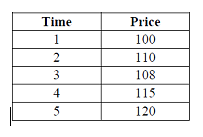The above time series data is reorganised as follows: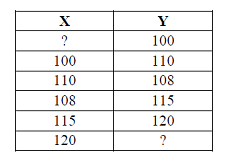It is seen that the previous time step is the input (X) and therefore the next time step is the output (Y) during this supervised learning problem. It is observed that there's no previous value that may be accustomed to predicting the primary value within the sequence. This row is deleted because it can't be used. Additionally, there's no known next value for the prediction of the last value within the sequence. This value is deleted while training the supervised model.

The window approach refers to the process of forecasting future time steps using data from earlier time steps. In some writing, it should simply be referred to as the window approach. This is also referred to as a lag or the lag technique in statistics and statistical analysis. We can convert any statistical dataset into a supervised learning issue using this window as the foundation. We'll explore how this may apply to supervised learning problems that use real-valued or labelled statistics and either regression or classification.

The first step that was performed was to fetch the values or download them as a CSV file. During this work, the stock prices of the Coca-Cola company within the date range 1-Jan-2017 to 1-Jan-2018 were obtained. The obtained data frame had two columns namely, Date and shut which were initially plotted onto the graph using the plot() functions. A linear model was later fit to this graph and displayed and observations were made as below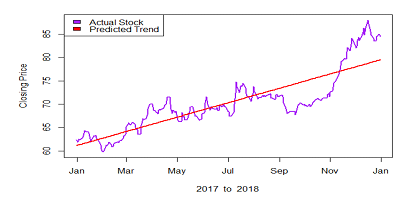The Support Vector Machine, which is classified as a Classification algorithm, is the other cutting-edge technology. A non-probabilistic binary linear classifier is created by an SVM training algorithm given a set of coaching instances, each of which has been labelled as belonging to one of two categories or the opposite. An SVM model might be a mapping of the instances as points in space with a visible gap as big as feasible between the examples of the various categories. The category to which a new example will belong is therefore determined by the gap into which it falls. Figure 1 depicts an SVM's visual details. The SVM classifies the points to the left and right of the support vectors into two distinct groups.

To learn more about Machine Learning the best place is 360DigiTMG, with multiple awards in its name 360DigiTMG is the Best place to start your Machine Learning Classes in Hyderabad. Enroll now!

The model produced by SVR depends only on a subset of the training data because the price function for building the model ignores any training data near the model prediction. A support vector machine as stated in this literature plots points on a hyperplane specified data points belonging to 2 different classes are separated by Support Vectors by the most important gap possible. But this is often defined for Classification problems which might be extended for Regression. It's therefore, conclusive that SVM performs better than LR. The resultant plots are shown below in the figures.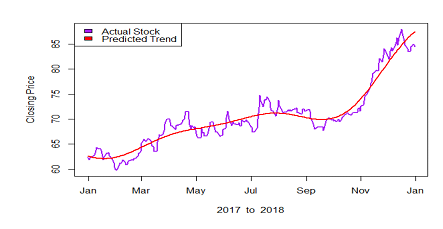The below are the Error Function of Linear Regression and Support Vector Machine respectively.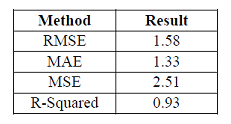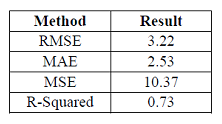Two ML methods may now be used to make predictions. Using the two strategies, each of which has benefits and drawbacks. Applying rectilinear regression to a sample of readily accessible data, conducting observations, and then using support vector regression to do more observations. The two strategies are compared after the observations and results are shown on a graph, and it is determined that the Classification model is appropriate for prediction in statistical data.

### Data Analyst Courses in Other Locations